# 概率论与数理统计示例（第一部分）：基础与初级理论

### 内容

1. 概述
2. 理论基础
3. 初级理论
• 3.1. 组合概率
• 3.2. 伯努利（Bernoulli）方案
4. 数理统计基础
5. 在初级理论框架内应用数理统计的示例
• 5.1. 参数点估值
• 5.2. 检验统计假设
6. 结束语
7. 附带文件

### 2. 理论基础

1) 样本空间是随机实验所有可能结果的集合。 它通常用大写的“欧米茄”希腊字母 – Ω 表示，并在图像上刻画为一个图形。 初级事件（采样点）通常用小写的 “欧米茄”希腊字母 − О 表示，并在图像上刻画为一个点。 描述抛硬币结果的最简单标准示例：Ω={ω1, ω2}，其中 ω1=H，且 ω2=T 表示硬币的正面或反面，而花括号表示其元素枚举给出的集合。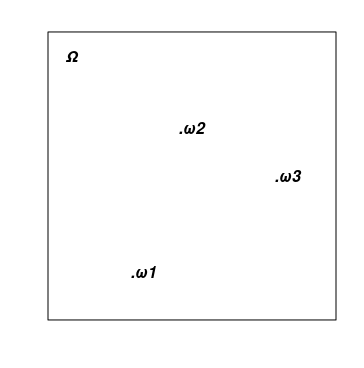2) 随机事件集合。 每个这样的事件都是一个初级事件集合（所有 Ω 基本事件的子集）。 事件集合包括一个空集 ∅={}（一个永远不会发生的事件），和整个 Ω 集（一个总会发生的事件）。 集合中两个事件的组合（和交集）也应属于集合。 在数理中，这样的集合通常称为集合代数。 上面的硬币例子有四个事件: {}=∅{H}, {T}{H,T}=Ω。 （自测问题：一个初级事件可以被视为随机事件的一个例子吗？）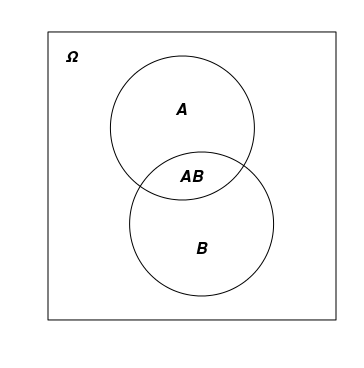3) 概率是一个数值函数 P=P(A) 匹配于实数范围从 01 的每个随机事件 AP(Ω)=1P(∅)=0。 此外，满足可加性规则：如果 A 事件是一个非重叠 BC 事件的组合，则 P(A)=P(B)+P(C)。 除“概率”项之外，P() 函数应用 “Ω 的概率分布”（或简单地说“Ω 分布”）。 不要把这个概念与类似的“随机变量分布函数”概念混淆。 它们彼此相关，但仍有区别。 前者是把一个数值与集合匹配的函数，后者只是一个数值与数值匹配的普通数值函数。

### 3. 初级理论

3.1. 组合概率

Ω完全由 N 初级结果组成，那么若包含它们中的 m 数量的结果，则它们的事件概率等于 m/N。 此处的概率计算包括选项数量在内。 作为规则，需用组合方法对付它，故此得名。 以下是一些示例：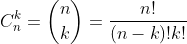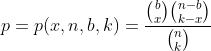• n, bk 假设为固定参数。
• 取代 Ω 样本空间，基于它建造一个新的 Ωх={0, 1, ..., k}。 新空间大概由 х 个值组成。
• 将每个 {х} 事件（由一个初级事件组成）与上面所示的超几何分布方程指定的概率相匹配。

3.2. 伯努利（Bernoulli）方案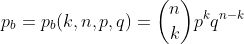• 基于伯努利方案的 Ω 样本空间，构建一个新的 − Ω1 仅包含单词，其中 H 精确出现了 b 次。
• 由于在 Ω1 当中的任意 A 事件也是 Ω 当中的一个事件，P(A) 即是为它定义的概率。 基于此事实，我们根据方程 P1(A)=P(A)/Р(Ω1)为 Ω1 引入 P1 概率。 事实上，这里用的是条件概率方程 P1(A)=P(A|О1)
• 现在研究来自 Ω1"一个长度为 k 的单词的后缀正好包含 x 个 H 个字母"的事件概率 P1()。 结果表明，这个概率正是由上面提供的超几何分布方程设定的。 非常值得注意的是，方程不影响 p 参数。

### 5. 在初级理论框架内应用数理统计的示例

5.1. 参数点估值

```from scipy.stats import hypergeom

n = 1000
k = 20
x = 1
lhx = 0.0
be = 0
for b in range(x, n - k + x):
lh = hypergeom.pmf(x, n, b, k)
if lh > lhx:
be = b
lhx = lh

print("be =",be)

```

```from scipy.stats import hypergeom

b = 50
k = 55
x = 3
lh0 = 0.0
ne = b + k - x - 1
while True:
lh = hypergeom.pmf(x, ne+1, b, k)
if lh < lh0:
break
else:
lh0 = lh
ne += 1

print("ne =",ne)

```

```// 构造走势的离散 mv[] 数组
// 以指定的百分比步长，在指定的时间区间
void setmv(int& mv[], datetime t1, datetime t2, double dpr)
{
int ND = 1000;
ArrayResize(mv, 0, ND);
// 获取价格历史记录
double price[];
int nprice = CopyOpen(Symbol(), PERIOD_M1, t1, t2, price);
if(nprice < 2)
{
Print("not enough price history");
return;
}
// 构造 mv[]
int lvl = 0, dlvl, nmv = 0, dmv;
double lp0 = log(price), lstep = log(1 + 0.01 * dpr);
for(int i = 1; i < nprice; ++i)
{
dlvl = (int)((log(price[i]) - lp0) / lstep - lvl);
if(dlvl == 0) continue;
lvl += dlvl;
dmv = 1;
if(dlvl < 0)
{
dmv = -1;
dlvl = -dlvl;
}
ArrayResize(mv, nmv + dlvl, ND);
for(int j = 0; j < dlvl; ++j) mv[nmv + j] = dmv;
nmv += dlvl;
}
}

```

discret_prices.mq5 脚本显示的结果为 1 和 -1 的序列，以及起始价格的离散模拟图。

```#include <Discr.mqh>
#include <Graphics\Graphic.mqh>
#property script_show_inputs
//+------------------------------------------------------------------+
input datetime tstart = D'2020.05.20 00:00';  // 所研究时间区间的开始
input datetime tstop = D'2020.06.20 00:00';   // 所研究时间区间的结束
input double   dprcnt = 0.5;                  // 价格离散化步长百分比
//+------------------------------------------------------------------+
void OnStart()
{
int mv[], nmv;
setmv(mv, tstart, tstop, dprcnt);
nmv = ArraySize(mv);
if(nmv < 1)
{
Print("not enough moves");
return;
}
// 显示 mv[] 为 1 和 -1 的序列
string res = (string)mv;
for(int i = 1; i < nmv; ++i) res += ", " + (string)mv[i];
Print(res);
// 显示 mv[] 累加汇总图表
ChartSetInteger(0, CHART_SHOW, false);
CGraphic graphic;
graphic.Create(0, "G", 0, 0, 0, 750, 350);
double x[], y[];
ArrayResize(x, nmv + 1);
ArrayResize(y, nmv + 1);
x = y = 0.0;
for(int i = 1; i <= nmv; ++i)
{
x[i] = i;
y[i] = y[i-1] + mv[i-1];
}
ArrayPrint(x);
ArrayPrint(y);
graphic.CurvePlotAll();
graphic.Update();
Sleep(30000);
ChartSetInteger(0, CHART_SHOW, true);
graphic.Destroy();
}

```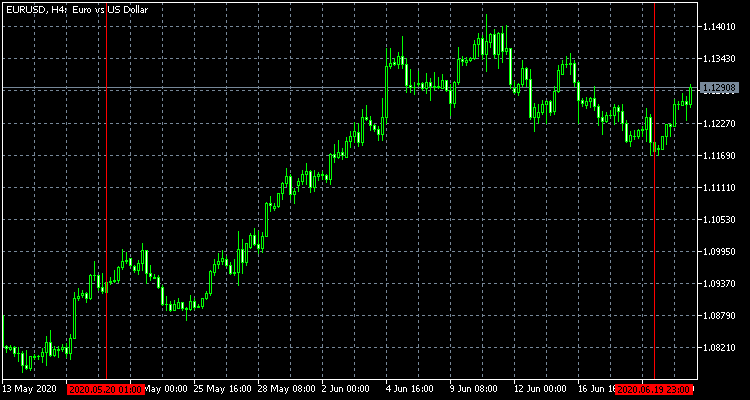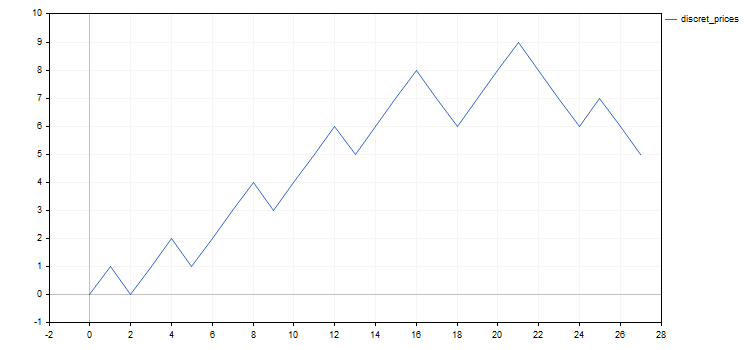1. 交易结果中，成交带有固定的的止损和止盈，及交易量等级。 一系列交易的所有利润大致相等，亏损也是如此。 故此，盈利概率是描述交易结果的一个充分参数。 例如，我们可以提出一个问题，即在现有的成交序列中，盈利的概率是否足够高。
2. 选择要投资的资产。 假设我们在区间伊始有大量的投资方法可供选择。 其中任何一个，都有一定的概率，最终都可能导致投资基金的彻底亏损。 如果有选择资产的方法，那么可能提出疑问：所择资产与规避资产之间最终导致破产概率的差异。

我们回到示例。

示例 3. 估算伯努利方案中的 p 参数。 这是罕见案例，可以在纸上解决的问题。 似然函数如下：p^nup*(1-p)^ndn。 取 p 的导数，并令其等于零，获得估算 р 的期望值作为频率: pe=nup/(nup+ndn)=nup/nmv。 通常情况下，执行的搜索不是针对似然函数的最大值，而是针对其对数，这要容易得多。 答案是一样的，因为对数是严格递增的函数，并且在相同的参数值下达到最大值。 以下是计算估值的 p_model.mq5 脚本。

```#include <Discr.mqh>
#property script_show_inputs
//+------------------------------------------------------------------+
input datetime tstart = D'2020.05.20 00:00';  // 所研究时间区间的开始
input datetime tstop = D'2020.06.20 00:00';   // 所研究时间区间的结束
input double   dprcnt = 0.5;                  // 价格离散化步长百分比
//+------------------------------------------------------------------+
void OnStart()
{
int mv[];
setmv(mv, tstart, tstop, dprcnt);
if(ArraySize(mv) < 1)
{
Print("not enough moves");
return;
}
double pe = calcpe(mv);
Print("pe=", pe);
}
//+------------------------------------------------------------------+
// 计算概率估值
double calcpe(int& mv[])
{
int nmv = ArraySize(mv);
if(nmv < 1) return 0.0;
int nup = 0;
for(int i = 0; i < nmv; ++i) if(mv[i] > 0) ++nup;
return ((double)nup) / nmv;
}

```

答案: pe = 0.59 (四舍五入)

示例 4. 伯努利方案修订版的参数估值。 正如我在上面所写的，伯努利方案可以很好地根据我们的建模目的进行更改。 我们来研究一个可能的修改选项。

也许，最简单的选择将走势序列切分成两个更小的序列，一个接着一个，并与伯努利方案及其参数相对应: n1, p1, n2=n-n1p2，其中 n 是累积序列的长度。
因此，需要估算三个参数 n1, p1p2。 最大化对数似然函数 p1p2，令我们可以通过 n1 来分析表达它们。 n1 的估值可简单地将 p1 和 p2 代入对数似然方程求解得到。 下面是计算参数估值的 p1p2_model.mq5 脚本。

```#include <Discr.mqh>
#property script_show_inputs
//+------------------------------------------------------------------+
input datetime tstart = D'2020.05.20 00:00';  // 所研究时间区间的开始
input datetime tstop = D'2020.06.20 00:00';   // 所研究时间区间的结束
input double   dprcnt = 0.5;                  // 价格离散化步长百分比
//+------------------------------------------------------------------+
void OnStart()
{
int mv[];
setmv(mv, tstart, tstop, dprcnt);
if(ArraySize(mv) < 2)
{
Print("not enough moves");
return;
}
double p1e, p2e;
int n1e;
calc_n1e_p1e_p2e(mv, n1e, p1e, p2e);
Print("n1e=", n1e, " p1e=", p1e, " p2e=", p2e);
}
//+------------------------------------------------------------------+
// 计算概率估值
void calc_n1e_p1e_p2e(int& mv[], int& n1e, double& p1e, double& p2e)
{
n1e = 0;
p1e = p2e = 0.0;
int nmv = ArraySize(mv);
if(nmv < 2) return;
n1e = 1;
double llhx = llhx_n1(mv, 1, p1e, p2e), llh, p1, p2;
for(int n1 = 2; n1 < nmv; ++n1)
{
llh = llhx_n1(mv, n1, p1, p2);
if(llh > llhx)
{
llhx = llh;
n1e = n1;
p1e = p1;
p2e = p2;
}
}
}
//+------------------------------------------------------------------+
// 对数似然函数最大值取决于 n1
double llhx_n1(int& mv[], int n1, double& p1, double& p2)
{
p1 = p2 = 0.0;
int nmv = ArraySize(mv);
if(nmv < 2 || n1 < 1 || n1 >= nmv) return 0.0;
int nu1 = 0, nu2 = 0;
for(int i = 0; i < n1; ++i) if(mv[i] > 0) ++nu1;
for(int i = n1; i < nmv; ++i) if(mv[i] > 0) ++nu2;
double l = 0.0;
if(nu1 > 0)
{
p1 = ((double)nu1) / n1;
l += nu1 * log(p1);
}
if(nu1 < n1) l += (n1 - nu1) * log(((double)(n1 - nu1)) / n1);
if(nu2 > 0)
{
p2 = ((double)nu2) / (nmv - n1);
l += nu2 * log(p2);
}
if(nu2 < nmv - n1) l += (nmv - n1 - nu2) * log(((double)(nmv - n1 - nu2)) / (nmv - n1));
return l;
}

```

答案: n1e = 21; p1e = 0.71; p2e = 0.17 (数字四舍五入)。 这似乎很明显 我们的模型行情的末尾“检测”到价格方向的变化（或调整）。 这提供了建议，针对这种情况，过渡到更复杂的模型并非徒劳。

示例 5. 在前面的示例中，走势上移的概率取决于它的数字（在时间上）。 我们来研究一下伯努利方案的另一个修订版，其中这个概率取决于之前的走势本身。 我们来构造一个模型，它是最简单的两个状态 Markov 链 的一个例子。

假设给走势编号，从 0n。 若走势为零，走势上移的概率简单地设置为等于 0.5，因为没有以前的走势。 对于其他位置，如果之前的走势向上，上移的概率等于 p1；如果之前的走势是向下的话，则为 p2。 在这两种情况下走势向下的概率等于 q1=1-p1，相应地 q2=1-p2。 例如，一个事件有一个初级事件的概率 "向下-向上-向上-向下"0.5*p2*p1*q1

我们已得到了一个含有两个参数的模型，可以用极大似然估值。 此处的所有计算都可以在纸上进行，特别是当我们最大化对数似然函数时。 与伯努利方案一样，答案归结为频率。 上行走势之后发生下行走势的次数可设为 nud (u 上行, d 下行)。 以类似的方式，引入 nuu, nddndu。 估算 p1p2 可由 p1ep2e 表示。 然后利用这些方程得到这些估值 p1e=nuu/(nuu+nud)p2e=ndu/(ndu+ndd)

下面是计算这些估值的 markov_model.mq5 脚本。

```#include <Discr.mqh>
#property script_show_inputs
//+------------------------------------------------------------------+
input datetime tstart = D'2020.05.20 00:00';  // 所研究时间区间的开始
input datetime tstop = D'2020.06.20 00:00';   // 所研究时间区间的结束
input double   dprcnt = 0.5;                  // 价格离散化步长百分比
//+------------------------------------------------------------------+
void OnStart()
{
int mv[];
setmv(mv, tstart, tstop, dprcnt);
if(ArraySize(mv) < 2)
{
Print("not enough moves");
return;
}
double p1e, p2e;
calcpes(mv, p1e, p2e);
Print("p1e=", p1e, " p2e=", p2e);
}
//+------------------------------------------------------------------+
// 计算概率估值
void calcpes(int& mv[], double& p1e, double& p2e)
{
p1e = p2e = 0;
int nmv = ArraySize(mv);
if(nmv < 2) return;
int nuu = 0, nud = 0, ndu = 0, ndd = 0, nu, nd;
for(int i = 0; i < nmv - 1; ++i)
if(mv[i] > 0)
{
if(mv[i + 1] > 0) ++nuu;
else ++nud;
}
else
{
if(mv[i + 1] > 0) ++ndu;
else ++ndd;
}
nu = nuu + nud;
nd = ndu + ndd;
if(nu > 0) p1e = ((double)nuu) / nu;
if(nd > 0) p2e = ((double)ndu) / nd;
}

```

答案:  p1e = 0.56; p2e = 0.60 (数字四舍五入)。 两个概率几乎相等，表明相邻走势之间没有相关性。 两个概率都大于 0.5 ，表明此为上升趋势。 两个概率估值都接近于一个更简单模型的概率估值（第三个例子中的简单伯努利方案），这表明在这种特殊情况下，过渡到更复杂模型毫无必要。

5.2. 检验统计假设

在同一样本空间上可以设置不同的概率分布。 猜想是指定分布类型的陈述。 应该有几个猜想（至少两个），因为解决方案应建立在一个猜想优于其他。 如果一个猜想即可唯一断定分布类型，就称之为简单猜想。

我们提供伯努利方案的例子：p 参数等于某个数值  猜想 − 一个简单猜想（只有一个可能的参数值，明确定义了分布），而参数超过指定值的猜想是一个复杂的猜想（可能的参数值是无限的）。

接下来，我们将讨论如何选择两个猜想中的一个。 其中一个称为 null，另一个备选的。 猜想是用一个统计标准来选择的。 基本上，这只是一个在样本空间内含有 01 值的函数，其中 0 意味着接受空猜想，而 1 表示接受备选。

因此，我们既可以接受空猜想，也可以拒绝它。 相应地，备选猜想要么被拒绝、要么被接受。 每一个决定都可能是对的、或是错的。 因此，接受这个猜想有四种可能的结果，其中两种是正确的，两种是错误的。

1. 类型 1 错误 − 空猜想的错误否定
2. 类型 2 错误 − 空猜想的错误接受

第 1 类和第 2 类错误的概率由 a1a2 表示。 很自然，最好尽可能减少这些可能性。 但不幸的是，这是不可能的。 甚至，通过降低某种类型错误的概率，我们相当期待面临另一类错误发生概率的增加。 这就是为什么通常要在这些错误之间做出某种妥协。

1-a1 被称为测试意义，而 1-a2 叫做它的动力。 测试动力很难计算，尤其是对于复杂的猜想。 因此，通常只用到测试意义。

标准通常基于一个称为检验统计量的数值函数（也定义为基于样本空间）。 它的多个值分为两个区域。 其中一个区域对应于接受空猜想，而另一个区域对应于拒绝。 拒绝空猜想的区域称为临界区。

下面是关于“负优先”猜想的一个重要注释。 这个条件意味着我们应该事先确保两个猜想中的一个得以满足。 粗略地说，如果我们把吉娃娃和其他品种的狗按重量分开，那么我们必须确保我们的天平上总有一只狗（不是大象、老鼠或猫）。 否则，我们的测试就没有意义了。 当计量经济学中所应用的统计条件不准确时，这一原则最常被违反。

下面是伯努利方案检验猜想的例子。

示例 1. 在伯努利方案里检验空猜想 p=p0。 这个猜想很简单。 备选猜想可以是以下两种选项之一（这两种情况都很复杂）。 kup （序列中上行走势的次数）被用作两种情况下的统计标准，尽管临界区域的定义不同。

1. p>p0，临界区域在右边 kup⋝kr
2. p<p0，临界区域在左边 kup⋜kl

krkl 的具体值可按条件查找，统计概率落入临界区域不会超过所选的 1 类错误概率。 正如我已经说过的，所有这些概率都是依据条件计算出来的，此刻满足空猜想（伯努利分布的参数 p=p0)。

我们在同样的离散价格序列上检验这个猜想，即 p0=0.5p0 的值很大，因为它符合“随机游走”（“公平硬币”）猜想。

下面的这个脚本 p0_hypothesis.mq5 验证这个猜想。

```#include <Math\Stat\Binomial.mqh>
#property script_show_inputs
//+------------------------------------------------------------------+
input int    nm = 27;          // 序列中的走势总数
input int    kup = 16;         // 上行走势的数量
input double p0 = 0.5;         // 检查概率值
input double alpha1 = 0.05;    // 类型 1 错误概率
//+------------------------------------------------------------------+
void OnStart()
{
int kl, kr, er;
kr=(int)MathQuantileBinomial(1.0-alpha1,nm,p0,er);
kl=(int)MathQuantileBinomial(alpha1,nm,p0,er);
Print("kl=", kl, " kup=", kup, " kr=", kr);
Print("kup<=kl = ", kup<=kl);
Print("kup>=kr = ", kup>=kr);
}

```

回应：

• kl=9; kup=16; kr=18
• kup<=kl = false
• kup>=kr = false

结果表明，在这种对于任何备案均有可能的明显价位上，空猜想不能被拒绝。

示例 2. 假设我们有两个长度为 n1n2 的序列，它们取自两个参数为 p1p2 的伯努利方案。 此处的空假设是在于满足 p1=p2 等式，而可能的备选方案是不同类型的不等式，这里也有两种。 k2 作为所有这些情况下的统计标准 − 两个序列中第二个序列中上行走势的次数，尽管临界区域的定义不同。

• p2>p1，临界区域在右边 k2⋝kr
• p2<p1，临界区域在左边 k2⋜kl

与前一个例子的显著区别，此处我们讨论的并非关于 p1p2 数值的精度，而是关于它们的比率。 临界区域的定义方法与前一个例子相同，但在空猜想下，统计分布是超几何的，而不是二项式的。 在数理统计中，这个标准被称为费舍尔精确检验。

下面是用 Python 编写的解决这个问题的 p1p2_hypothesis.py 脚本，将离散化的价格一分为二。 我把来自上一段落中第四个示例中获得的数据拆分。

```from scipy.stats import hypergeom

n1 = 21; n2 = 6
k1 = 15; k2 = 1
alpha1 = 0.05

kl = int(hypergeom.ppf(alpha1, n1 + n2, k1 + k2, n2))
kr = int(hypergeom.ppf(1.0 - alpha1, n1 + n2, k1 + k2, n2))

print("kl =", kl, " k2 =", k2, " kr =", kr)
print("k2<=kl =", k2<=kl)
print("k2>=kr =", k2>=kr)

```

回应：

• kl = 2;  k2 = 2;  kr = 6
• k2<=kl = True
• k2>=kr = False
结果表明，在这个明显有利于第一备案的价位上，空猜想被拒绝。 这是一个额外的确认，我们的价格部分可以分成朝不同方向移动的两部分。

### 6. 结束语

本文并未涉及到数理统计的重要领域，如描述性统计、区间参数估值、渐近估值和统计学中的贝叶斯方法。 原因是我需要澄清随机变量的概念，从而对其进行有意义的研究。 我决定在下一篇文章中再做这件事，从而令本篇幅足够紧凑。

### 7. 附件

#
名称
类型 说明
1 hyperg_be.py   Python 脚本  计算 b 超几何分布参数估值。 示例 5.1.1
2 hyperg_ne.py   Python 脚本   计算 n 超几何分布参数估值。 示例 5.1.2
3  Discr.mqh  MQL5 头文件  在所有 MQL5 示例中价格离散化均用到的 setmv()函数文件
4  discret_prices.mq5  MQL5 脚本  执行价格离散化，并以 1-1 序列形式显示结果图表的脚本
5  p_model.mq5  MQL5 脚本  计算伯努利方案中的 p 参数估值。 示例 5.1.3
6  p1p2_model.mq5  MQL5 脚本  计算由两个伯努利方案组成的模型中 n1, p1p2 参数估值。 示例 5.1.4
7  markov_model.mq5  MQL5 脚本  计算 Markov 链中的 p1p2 参数估值。 示例 5.1.5
8  p0_hypothesis.mq5  MQL5 脚本
检验伯努利方案猜想的参数是否等于指定数字。 示例 5.2.1
9  p1p2_hypothesis.py  Python 脚本  费舍尔精确检验。 示例 5.2.2

## 量化课程

移动端课程